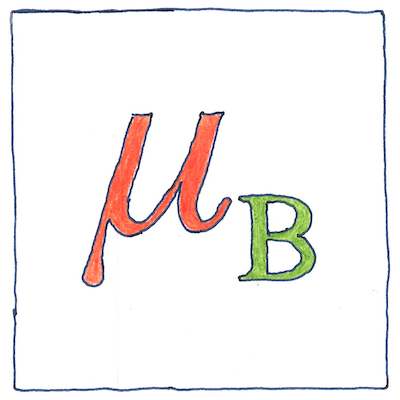# 1913

## The book of science

Tom Sharp

 Niels Bohr electromagnetism

## Bohr magneton

• The Bohr magneton is the magnitude
• of the magnetic dipole moment
• of an electron in a circular orbit
• with an orbital angular momentum
• equal to the reduced Planck constant.
• The Bohr magneton is not merely
• the natural unit of magnetism,
• but a physical constant,
• like the speed of light,
• that characterizes our universe.

## Its value

• The value of the Bohr magneton
• is related to Bohr’s model of the atom.
• It measures the magnetic moment
• of a single electron orbiting an atom.
• It is the electric charge of a proton
• times the Planck constant divided by 2π
• divided by 2 times the electron rest mass
• times the speed of light.

## Constants

• Universal constants
• Electromagnetic constants
• Atomic and nuclear constants
• Physico-chemical constants
• To understand how things work,
• we construct a theoretical model
• and then establish that all our measurements
• can be constructed from fundamental values.
• We hold these values to be constant
• for all time, everywhere in the universe,
• unlike mathematical constants like π,
• unlike desire, or free will.
• True idealists
• are not Platonic philosopers,
• not even young lovers,
• but theoretical physicists.

The Bohr magneton is based on the Bohr model of the atom, whose principles survived the development of quantum mechanics, even though it turns out that electrons do not have circular orbits. It worked fine to explain the hydrogen atom, but a more complex model was needed to explain heavier elements. An electron does not orbit the nucleus like a planet orbits the sun but behaves like a wave.